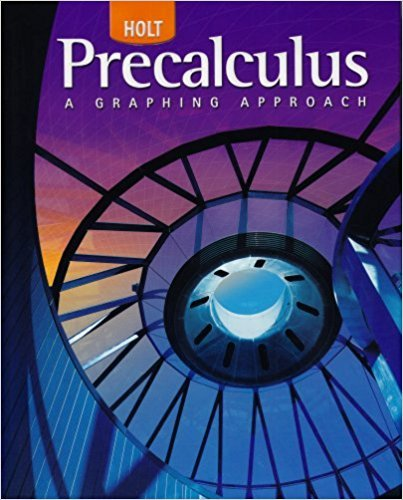×
×

# Solutions for Chapter 8.4.A: Excursion: Sound Waves## Full solutions for Precalculus | 1st Edition

ISBN: 9780030416477Solutions for Chapter 8.4.A: Excursion: Sound Waves

Solutions for Chapter 8.4.A
4 5 0 324 Reviews
29
1
##### ISBN: 9780030416477

Since 6 problems in chapter 8.4.A: Excursion: Sound Waves have been answered, more than 23915 students have viewed full step-by-step solutions from this chapter. Precalculus was written by and is associated to the ISBN: 9780030416477. Chapter 8.4.A: Excursion: Sound Waves includes 6 full step-by-step solutions. This textbook survival guide was created for the textbook: Precalculus, edition: 1. This expansive textbook survival guide covers the following chapters and their solutions.

Key Calculus Terms and definitions covered in this textbook
• Angle of elevation

The acute angle formed by the line of sight (upward) and the horizontal

• Bounded below

A function is bounded below if there is a number b such that b ? ƒ(x) for all x in the domain of f.

• Complex fraction

See Compound fraction.

• Control

The principle of experimental design that makes it possible to rule out other factors when making inferences about a particular explanatory variable

• Deductive reasoning

The process of utilizing general information to prove a specific hypothesis

• Difference identity

An identity involving a trigonometric function of u - v

• Horizontal translation

A shift of a graph to the left or right.

• Inverse function

The inverse relation of a one-to-one function.

• Law of sines

sin A a = sin B b = sin C c

• Lemniscate

A graph of a polar equation of the form r2 = a2 sin 2u or r 2 = a2 cos 2u.

• Limit

limx:aƒ1x2 = L means that ƒ(x) gets arbitrarily close to L as x gets arbitrarily close (but not equal) to a

• Midpoint (in Cartesian space)

For the line segment with endpoints (x 1, y1, z 1) and (x2, y2, z2), ax 1 + x 22 ,y1 + y22 ,z 1 + z 22 b

• Multiplication property of equality

If u = v and w = z, then uw = vz

• Product of complex numbers

(a + bi)(c + di) = (ac - bd) + (ad + bc)i

The distance from a point on a circle (or a sphere) to the center of the circle (or the sphere).

• Repeated zeros

Zeros of multiplicity ? 2 (see Multiplicity).

• Solve graphically

Use a graphical method, including use of a hand sketch or use of a grapher. When appropriate, the approximate solution should be confirmed algebraically

• Spiral of Archimedes

The graph of the polar curve.

• Symmetric property of equality

If a = b, then b = a

• Xscl

The scale of the tick marks on the x-axis in a viewing window.

×